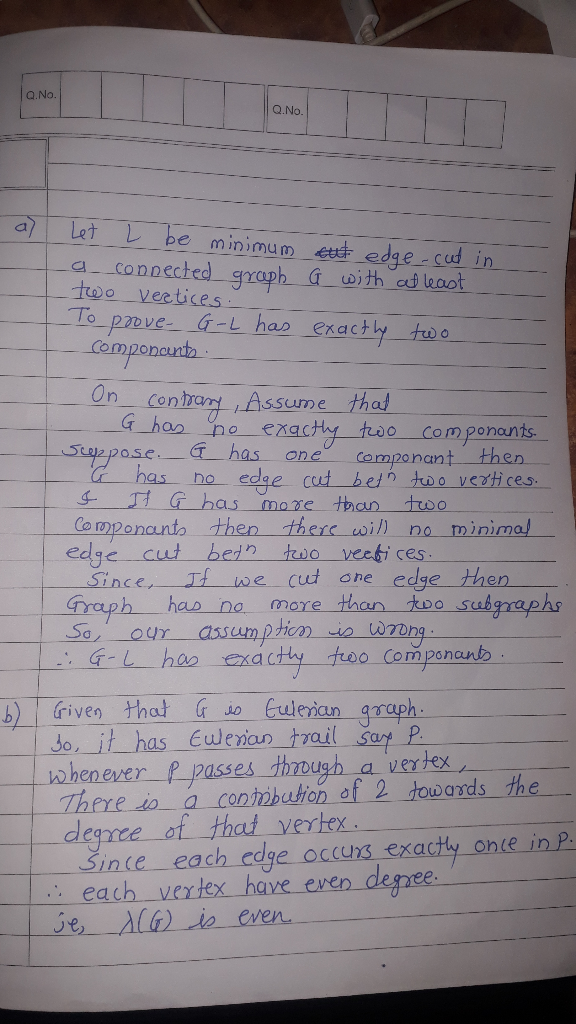# (a) Let L be a minimum edge-cut in a connected graph G with at least two vertices. Prove that G −...

(a) Let L be a minimum edge-cut in a connected graph G with at least two vertices. Prove that G − L has exactly two components.

(b) Let G an eulerian graph. Prove that λ(G) is even.##### Add Answer of: (a) Let L be a minimum edge-cut in a connected graph G with at least two vertices. Prove that G −...
More Homework Help Questions Additional questions in this topic.